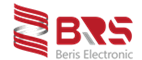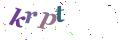English
Article

# Solenoid Valve Coil identification and basic knowledge on design

Solenoid Coils

Beris Coils are designed and tested for continuous service. They all meet the thermal endurance specifications according to IEC 216.

Coil’s own temperature rise due to energization.

Including effect of fluid temperature at catalogue rated limits (Electrical characteristics, solenoid operator’s ambient temperature range)

The standard coils are available for insulation classes E, F and H. The insulation class determines the coil’s maximum operating temperature for a specific life:

- Class H: 30 000 hours
- Class F: 20 000 hours

The temperature rise of continuously energized coils depends on size and power consumption .This, in turn, determines the maximum differential pressure rating of a valve as indicated in the catalogue.

An example for insulation class F. The insulation is designed for the coil to be operated at temperatures in accordance with class F, i.e. 155℃.The max. temperature rise of the coil when energized is limited, depending on the type of coil(e.g.80 ℃(FT),95℃,105℃(FB),130℃(FF)).

According to the type of coil used, the solenoid operator’s maximum ambient temperature (given under “Electrical characteristics” in the specific catalogue pages) including fluid temperature effects may be 75,60,50, or 25℃.

Determining factors may be either:

a) Temperature considerations (own temperature rise)
b) Power considerations
c) Higher temperature rise, as result of increased wattage (required for valve pressure ratings).

Calculations

For direct acting solenoids we can calculate the solenoid pull force by means of the following rough equation:

Fs = P.A (N)
Fs = solenoid pull-force (N)
P = pressure (Pa) (10 5 Pa=1bar)
A= orifice area (㎡)

Example

An average solenoid will have a pull force of approx.15N. To use this solenoid on a pressure differential of 1 MPa (10bar), we can calculate the maximum orifice diameter.

Fs = p.A → 15=106.A
A = 1,5.10-5 ㎡
A = 1/4 . π. d2 → d = 4.4mm

For low pressure applications such as gas burners, automatic dispensing or low vacuum systems up to 0.1 MPa the Orifice diameter equals to 19.5mm.

The internal pilot-operated constructions (floating diaphragm or floating piston) use a small orifice (the pilot) to control the pressure to the diaphragm or piston. Large main orifices can be opened or closed at reasonable pressure up to 15MPa.

Basic Design Considerations

The electrical field

To use solenoid as a driver for valves we have to learn first how the magnetism, generated by the solenoid, can be converted into mechanical energy.

If a certain voltage is applied to the coil of the solenoid an electrical current will flow through its windings and creates a magnetic field around the coil.

This filed depends on the amount of current, number of windings and length of the coil and be expressed by the following equation:

We discover, However, that the conductance of magnetic field –lines differs for all kind of material.

This conductance is called: permeability “μ”.

For vacuum the permeability:

u0=4. π.10-7  (H/m) or (Vs/Am)

u=u0..ur.[u=B/H]

ur air=1

We distinguish:

- diamagnetical:

ur < 1 (bismuth, antimony)

-paramagnetical:

ur =1 (aluminium ,copper)

-ferromagnetical :

ur> 1(iron,nickel,cobalt)

To identify the proper “ur” or induction “B” we can make use of the

so-called hysteresis-cycle-curves for the ferromagnetical materials.

BERIS’ core and plugnut material is special chemical high compatible ferromagnetical stainless steel. If tables are used, the following equation should be applicable:

B = u0..ur.H(T)

AC  and DC Solenoids

As it is important to know the electrical field we have to know therefore the current through the coil.

For DC constructions we can easily calculate the current with the equation:

However, For AC constructions we have not only to deal with pure ohmical resistance, but also with AC-resistance , the so-called reactance ‘xL’.

To find the impedance ‘Z’ we have to combine the ‘xL’ and ‘R’ values in a vector diagramme. Now we can calculate the current by :

The ‘xL’ value depends on the air gas between core and plugnut and is smaller when the gas is big.

Therefore we can find a difference between the current through the coil when the core is in its lower position (inrush) and a current with the core in its upper position (holding).

Pull force of a magnet

With the knowledge of the electrical field and induction we can determine the solenoid driver force by means of the following equation:

As the three graphs on the left show, the air gap between core and plugnut determines the induction “B” and therefore the pull force “F”, The so called pull-stroke curves do show for each solenoid their typical curves.

H  =  Magnetic field strength  (A/m)
I   =   Electrical current         (A)
N =   Number of turns          (1)
B = Magnetic flux density    (T)
U0 = Permeability of vacuum (H/m)
UR = Relative permeability   (1)
A   = Area of core                   (m2)
C=   Constant

Differences between AC and DC solenoids

There are two solenoid valve categories: The first category with identical AC and DC design offers easy adaption of the same valve to AC or DC; full interchangeability is ensured for alternating or direct current .

The Plugnut and core of the valves in the second category are conically shaped (choked) and a special non-magnetisable part has to be assembled to prevent sticking of the core in its upper position due to the presence of residue magnetism. This part is called a “breaker piece”

AC/DC Solenoid comparison

AC service

a) High inrush and low holding current
b) High pull force
c) Sensitive to dirt
d) The coils have less windings(copper) than DC coils
e) Power consumption and pull force not sensitive to temperatures.

DC service

a) Inrush current equals to holding current
b) Power consumption and pull force depend on temperature
c) Solenoid operates quietly
d) Not sensitive to dirt
e) The coils have more windings (copper) than AC coils

Power consumption for AC:

With:

PA = inrush VA value

PM = holding VA value

Power consumption for DC

P(W) = U.I

I(A) =  P(w)/U(V)

Nominal Power Ratings

The nominal power ratings indicated in the “ELECTRICAL CHARACTERISTICS” tables are average values and are based on measurements on standard products. In most cases the tables show values for cold and hot conditions.

The definitions below have to be recognized.

Cold nominal power ratings

This value in watts represents the amount of power dissipated by the coil system after connection to the power supply.

In this case the coil has the same temperature as the ambient or, in some situations, the temperature of the medium handled. The above results in a nominal cold coil resistance. The cold coil resistance is lower than the hot coil resistance thus resulting in a higher power rating under cold condition than under hot.

In practice the cold nominal power rating has to be taken into account when a solenoid valve is operated ( for the first time).

Hot nominal power ratings

After a certain period of time the coil system is warmed –up and reaches a final operational temperature. This period of time may differ, depending on solenoid construction, voltage variation, ambient temperature, valve size and piping system, from 1 to 5 hours of operation.

The above results in a nominal hot coil resistance.The hot coil resistance is higher than the cold coil resistance thus resulting in a lower power rating under hot condition than under cold.

In practice the hot nominal power rating has to be taken into account when calculations are to be carried out for cost or lifetime battery applications.

General remarks

The cold and hot power ratings are defined under nominal operation conditions i.e.:

- Nominal supply voltage(Un)
- Ambient and medium temperature 20℃

Please note that the following may influence the values given:

- Supply voltage variation
- Ambient temperature
- Medium temperature
- The size and type of the piping system.
Submit Comment
People who viewed this item also viewed
Latest News
Latest Exhibtions
Contact Now
Name:
Email:  Email is wrong!
Message:  Message is wrong!
SUBMITNo.12 Longxing Road, Longguan Town, Ningbo, China 315166